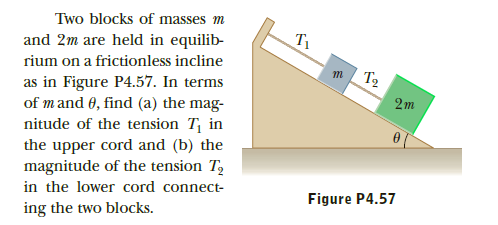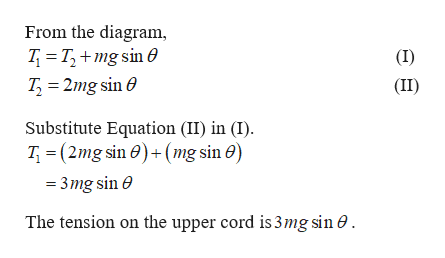# Two blocks of masses mT1and 2m are held in equilib-rium on a frictionless inclineT2as in Figure P4.57. In termsof mand 0, find (a) the mag-nitude of the tension T, inthe upper cord and (b) themagnitude of the tension T,in the lower cord connect-ing the two blocks.2mFigure P4.57

Question
1 viewshelp_outlineImage TranscriptioncloseTwo blocks of masses m T1 and 2m are held in equilib- rium on a frictionless incline T2 as in Figure P4.57. In terms of mand 0, find (a) the mag- nitude of the tension T, in the upper cord and (b) the magnitude of the tension T, in the lower cord connect- ing the two blocks. 2m Figure P4.57 fullscreen
check_circle

Step 1

The free body diagram is given below

Step 2

the tension on the upe...help_outlineImage TranscriptioncloseFrom the diagram, T = T,+mg sin O (I) T, = 2mg sin 0 (II) Substitute Equation (II) in (I). T, = (2mg sin 0)+(mg sin 0) = 3mg sin e The tension on the upper cord is 3mg sin 0 . fullscreen

### Want to see the full answer?

See Solution

#### Want to see this answer and more?

Solutions are written by subject experts who are available 24/7. Questions are typically answered within 1 hour.*

See Solution
*Response times may vary by subject and question.
Tagged in

### Newtons Laws of Motion